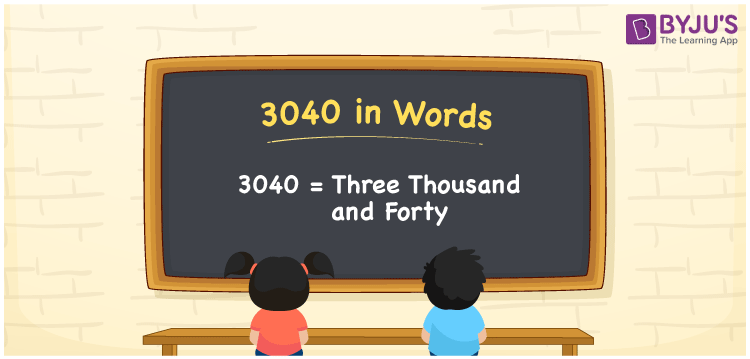# 3040 in Words

3040 in words can be written as Three Thousand and Forty. Students will be able to learn the conversion of 3040 in words which will help them understand the applications of numbers in our daily lives. If you buy a watch for Rs. 3040, then you can say that “I have bought a watch for Three Thousand and Forty Rupees”. The  number 3040 can be written in words using the English alphabet. The numbers in words can be grasped easily by the students using the resources given at BYJU’S. 3040 in English can be read as “Three Thousand and Forty”.

 3040 in words Three Thousand and Forty Three Thousand and Forty in Numbers 3040

## 3040 in English Words## How to Write 3040 in Words?

Students will learn about the conversion of 3040 into words from place value charts. The number 3040 has four digits. For 3040, the place value chart is prepared in a table form to help students understand it effectively.

 Thousands Hundreds Tens Ones 3 0 4 0

3040 in expanded form is explained in brief here:

3 × Thousand + 0 × Hundred + 4 × Ten + 0 × One

= 3 × 1000  + 0 × 100 + 4 × 10 + 0 × 1

= 3000 + 40

= 3040

= Three Thousand and Forty

Therefore, 3040 in words is written as Three Thousand and Forty.

3040 is a natural number that precedes 3041 and succeeds 3039.

3040 in words – Three Thousand and Forty

Is 3040 an odd number? – No

Is 3040 an even number? – Yes

Is 3040 a perfect square number? – No

Is 3040 a perfect cube number? – No

Is 3040 a prime number? – No

Is 3040 a composite number? – Yes

## Frequently Asked Questions on 3040 in Words

Q1

### How do you write 3040 in words?

3040 can be written as “Three Thousand and Forty” in words.
Q2

### Is 3040 an even number?

3040 is an even number because it is divisible by 2.
3040/2 = 1510
Q3

### How can Three Thousand and Forty be written in numbers?

Three Thousand and Forty can be written in numbers as 3040.